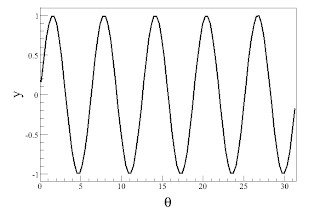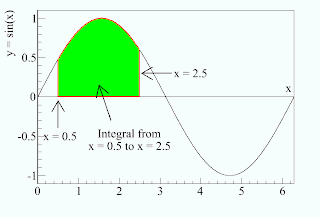## Friday, February 26, 2010

### Fourier analysis - Sines and integrals

In case anyone is still reading this, now that it is being updated so sporadically, I'm finally managing another post on Fourier analysis. In this post, I'll try to set up a little bit of the math behind the theory. To do so, I'm going to first remind everyone about the sine function, which I wrote about when talking about the Double Slit Experiment. In that post, I said that the sine function was a mathematical representation of a wave. Here is a plot of y = sin(θ):Now, that looks an awful lot like the sound waves I was looking at with my guitar back in November. Because they are the same. In fact, when I wanted to depict the sound waves graphically, I used the sine and its partner, the cosine to do it. Going back to the post on the double slit experiment, I believed I compared these functions to a part of speech; by using them, I can now describe a whole host of different phenomena that were previously inaccessible. Including sound waves.

Next, I want to talk about integrals. My mother never took calculus and says she has no idea what an integral is, which means I'm going to try to give a brief introduction (without going into details, alas). The first thing I was taught about integrals is that they represent the "area under the curve," and I think that's really all we need to know about them. If I draw a curve on a coordinate system, for example, like the sine curve above, then the integral is the area between the curve and the x-axis. Therefore, we need to know one other thing to define it, and that is the range of the integral. For example, I am going to zoom in slightly on that sine curve, and then I'll take the integral from x = 0.5 to x = 2.5, which is just the area below the curve between those limits, or the region shaded green.Now, things can get a bit trickier conceptually when the curve crosses the x-axis and becomes negative-valued. In this case, the integral is still the area under the curve, except that it is now negative. This is represented by the yellow shading.Finally, if you look carefully, you'll notice that the sine function appears to be symmetric. This will be really important for Fourier analysis. If you integrate the sign function over an entire period, the positive part and the negative part cancel each other out, and we're left with a total integral of 0.I want to make two final comments about integrals. The key to calculus is finding out that you can generally solve for these areas if you know the functional form of the curve (in this case, for example, we know the curve is a sine curve, so I could write down the function representing the area from calculus). And because I know this is the kind of thing that might interest my mom, in math, we represent an integral with a symbol that looks a little bit like an "S". For example, the integral of sin(x) is written like this:

$\int sin\left(x\right)dx$

The "dx" is there partly to let the reader know that the integral is being performed over the x variable.